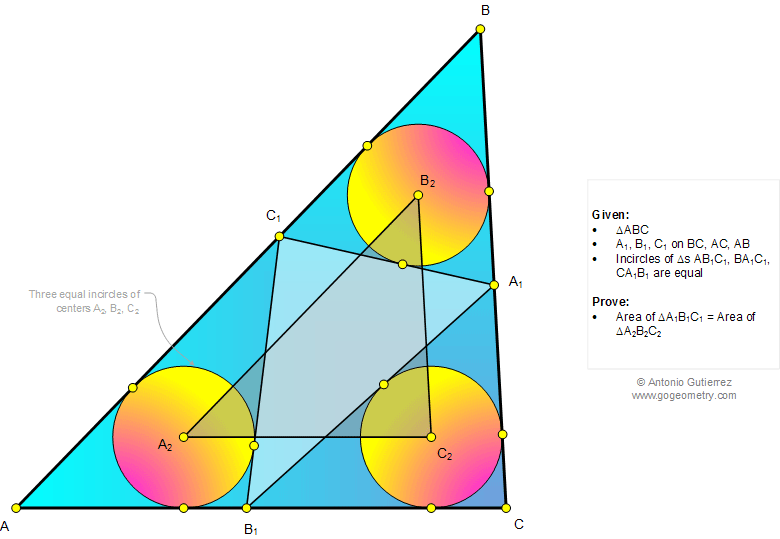# Online Math: Geometry Problem 1035: Triangle, Three equal Incircles, Tangent lines, Equivalent triangles, Equal area. Level: School, College.

< PREVIOUS PROBLEM  |  NEXT PROBLEM >

 The figure below shows a triangle ABC where A1, B1, and C1 are on BC, AC, and AB, respectively, so that the incircles of triangles AB1C1, BA1C1, and CA1B1 are equal. Prove that the areas of triangles A1B1C1 and A2B2C2 are equal (equivalent triangles).Home | SearchGeometry | Problems | All Problems | Open Problems | Visual Index | 10 Problems | Problems Art GalleryArt | 1031-1040 | Triangle | Circle | Incircle | Areas | Triangle area | Tangent Line | Email | by Antonio Gutierrez

Add a solution to the problem 1035
Last updated: Sep 19, 2014